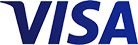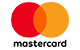Grid List
inc GST (Pair)
Increase value Decrease value
inc GST (Pair)
Increase value Decrease value
inc GST (Pair)
Increase value Decrease value
inc GST (Pair)
Increase value Decrease value
inc GST (Pair)
Increase value Decrease value
inc GST (Pair)
Increase value Decrease value
inc GST (Pair)
Increase value Decrease value
inc GST (Pair)
Increase value Decrease value
inc GST (Pair)
Increase value Decrease value
inc GST (Pair)
Increase value Decrease value
inc GST (Pair)
Increase value Decrease value
inc GST (Pair)
Increase value Decrease value